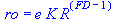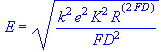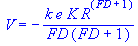FRACTAL BASICS A

The density ro, intensity Er and potential Vr of physical field in the distance r from the midpoint of the bounded area for

 Euclidean dimension:   E = 1 E = 2 E = 3 E = 4Programming code at Maple for calculation of quantities

 > restart: > with(linalg): > ED:=1; > X:=vector(ED): > r:=sqrt(multiply(X,X)): > V:=-k*e*K*r^(FD-ED+2)/(FD*(FD-ED+2)): > 'V'=subs(r=R,V); > EV:=grad(V,X): > E:=simplify(sqrt(multiply(EV,EV))): > 'E'=subs(r^2=R^2,E); > ro:=simplify(laplacian(V,X)): > 'ro'=subs(r^2=R^2,ro);